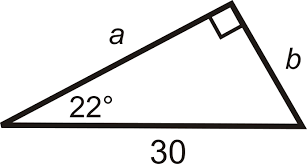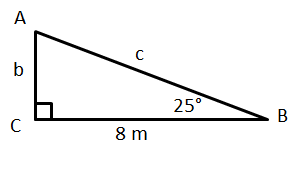# G.4.1,4-6 Part 4 Opener

Let's pratice the steps we learned in the last GeoGebra for finding a missing side of a triangle using a trigonometric ratio:
1. Label the side you're trying to find with x.
2. Use opposite, adjacent, or hypotenuse to label the side you know & the side you want to find.
3. Choose the trig ratio that involves the two sides you just labeled.
4. Set up an equation using the trig ratio you choose by filling in the angle and side length you know and x for the side you don't know (or if the unknown side is already labeled use that variable).
5. Solve the equation.

Use a trigonometric ratio to find side b. Give the equation you used to solve as well as the answer to b.Use a trigonometric ratio to find side AB. Give the equation you used to solve as well as the answer to AB.Use a trigonometric ratio to find side c. Give the equation you used to solve as well as the answer to c.